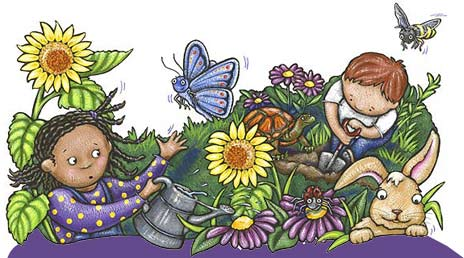Printer-friendlyartwork © Neecy TwinemPart of the AfterSchoolTreats.com family of creative enrichment websites!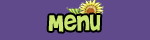HomeJoin Us!Garden CalendarKids' BlogRecipesLinks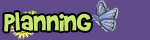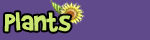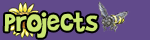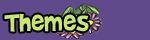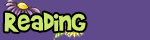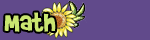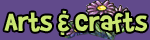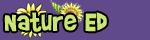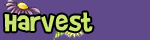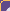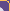Activities < Previous Next >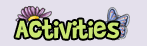Math: Fact Family Daisies Supplies: If you have them available, give each student a real daisy Yellow construction paper, one per student Paper plate | pencil White construction paper | petal pattern Scissors | Scotch tape Index cards with three one-digit numbers on each one (NOTE: this is four students in about fourth grade on up, but you can simplify it for younger children by including fewer petals and concentrating just on addition, for example.) Give each student a sheet of construction paper and let them trace around a paper plate to make a circle. Then should cut it out and draw a funny face on it. This will be the center of their "math daisy." Students should write their names on their daisy "face." (To save time, the leader might pre-cut 12 white construction paper "petals" for each student. Or you can give each student a petal pattern and have them trace and cut out 12 petals.)  Now give each student an index card and have them choose three single-digit (under 10) numbers to write on each card. They should be three different numbers, from 0 to 9. For example:  1     8     9 or 2      3      5 Students can use any combination of three single-digit numerals, though they should all be different. Now turn the students' attention to math fact families. Think of numbers as being like members of a great, big family. Math fact families are about relationships between numbers. Just two numbers, put together, create a "math fact family." But we're going to work with three numbers to create more relationships that we can explore. You can show these relationships of different math fact families by putting them into an equation. The equations can have to do with addition, subtraction, multiplication, or division. Then you can see how these numbers interact with each other, and with "relatives" - other numbers that can be brought into that math fact family by addition, subtraction, multiplication or division. We are going to create a daisy that shows the family relationships between the three numbers each student has written on their card. Now have each student write a different "fact" about the math relationships between those three numbers on their card. For example, the student whose three numbers were "1, 8, 9" could write "1 + 8 = 9" and "9 - 8 = 1.They should try to come up with at least 12 "fact families" for each index card. After you have checked their math fact families, they can copy each math fact on a petal, and tape the 12 petals to the back of the yellow daisy face. They should also write the three numbers on the bottom of the daisy face so they can remember how these numbers are related! For example, for the student who chose 1, 8 and 9, here are several fact families that could be written on the petals: 1 + 8 = 9 8 + 1 = 9 1 + 8 + 9 = 18 9 - 1 = 8 9 - 8 = 1 9 - 8 - 1 = 0 8 - 1 = 7 8 - 7 = 1 8 + 9 = 17 9 + 8 = 17 8 + 1 + 9 = 18 9 + 1 + 8 = 18 9 + 8 + 1 = 18 1 + 9 + 8 = 18 8 + 9 + 1 = 18 And here are examples for a student who chose 2 3 5: 2 + 3 = 5 3 + 2 = 5 5 - 2 = 3 5 - 3 = 2 3 + 5 = 8 5 + 3 = 8 2 + 5 = 7 3 - 2 = 1 2 + 3 + 5 = 10 2 + 5 + 3 = 10 3 + 2 + 5 = 10 3 + 5 + 2 = 10 5 + 2 + 3 = 10 5 + 3 + 2 = 10 At the end of this activity, tape the daisies on the board or send them home. Give each student a real daisy, and each student should do the traditional "He loves me, he loves me not. . . ." activity (or "she" if it's a male student!). They can pull off one petal for each statement. Whatever statement you're on when you come to the last petal (either "loves me" or "loves me not") is the magical "answer." But NOT to a mathematical certainty! By Susan Darst Williams  www.KidsGardenClub.org  'Rithmetic 01  © 2010
 Activities < Previous Next >^ return to top ^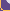Copyright ©2019 KidsGardenClub.org. All Rights Reserved.